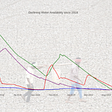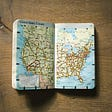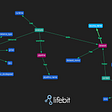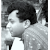Facebook Prophet: Time Series Prediction for Everyone

# Time series data model

Typically a time series has followingcomponents

1. A timestamp: To record when something has happened
2. metrics: To record quantity of the entity. It can be “price” of a ticker, or blood pressure at a given time.

It is easy to see time series data can be found almost every industry. And it is interesting to understand not only data points behaved in past, but also how it is going to behave in future. In general parlance it is called “Forecast”.

In this post, lets look at Prophet, a python (and R) library open sourced by Facebook. From Prophet’s website,

Prophet is a procedure for forecasting time series data based on an additive model where non-linear trends are fit with yearly, weekly, and daily seasonality, plus holiday effects. It works best with time series that have strong seasonal effects and several seasons of historical data. Prophet is robust to missing data and shifts in the trend, and typically handles outliers well.

In simple words, Prophet is a simple library which can be used by non-data-scientists, (almost) out of the box and still get a pretty reasonable forecasts.

So, first things first. Let’s get a dataset. I live in Melbourne, Australia. Hence I used Melbourne Pedestrian Sensor Data and Sensor Location Data. Once downloaded, you will have 2 pipe separated files

`import osfiles = [f for f in os.listdir(".") if f.endswith('.csv')]print(files)['Pedestrian_Counting_System_-_Sensor_Locations.csv', 'Pedestrian_Counting_System_-_Monthly__counts_per_hour_.csv']`

Now, Let’s import the data. And Start rolling. We will use just Alfred Plac e sensor’s data for this analysis.

`import pandas as pdimport numpy as npfrom fbprophet import Prophettdf = pd.read_csv("Pedestrian_Counting_System_-_Monthly__counts_per_hour_.csv")tdf = tdf[(tdf.Sensor_Name == 'Alfred Place') ]`

Now, let us prepare our dataframe for Prophet. Prophet needs input dtaframe to have columns named as “ds” for timestamp and “y” for metric. It is important to rename the columns like so. Also, ds must of date or datetime type.

`import matplotlib.pyplot as plttdf['ds'] = pd.to_datetime(tdf.Date_Time, format="%m/%d/%Y %I:%M:%S %p") tdf = tdf.sort_values(by=['ds'])tdf['y'] = tdf['Hourly_Counts']ddf_data = tdf[['ds', 'y']]ddf_data.set_index('ds').plot(style='.', figsize=(15,5), color='#00BA38', title='Pedestrian Counting System - Melbourne')plt.show()`

One thing to note that 2020 is terrible and has not even remotely comparable to previous years. We all now know why: COVID-19. So we will remove 2020 from our data completely.

Now, let us divide the data into test and training buckets. Due to its temporal nature, it is suggested to use timestamp to do the split. Lets split the data till 2018 for training and keep 2019 for testing.

`import datetimesplit_date = datetime.datetime(2018,12,31,0,0,0)ddf_train = ddf_data[(ddf_data.ds <= split_date)].copy().set_index("ds")ddf_test = ddf_data[(ddf_data.ds > split_date) & (ddf_data.ds < upto_date)].copy().set_index("ds")plt_dt = ddf_test \    .rename(columns={'y': 'test'}) \    .join(ddf_train.rename(columns={'y': 'training'}),          how='outer')plt_dt.plot(figsize=(15,5), title='Pred', style='.')plt.show()`

Believe it or not, we are all set for building our first Prophet model, and do some prediction with it.

`model = Prophet()model.fit(ddf_train.reset_index())forecast = model.predict(ddf_test.reset_index())`

YES!! It is that easy. Let us visualize the results.

Well, lots going on here. Let us understand it a bit. The black section is fairly easy to understand, it is the actuals from training dataset. Prophet is actually capable of filling in missing values, so it is handy to see the past and future in single plot. The blue section is forecast, and darker blue section is with 95% confidence.

But, wait, it is terrible. It forecasts negative pedestrian counts, which makes no real sense. Lets look a bit closer. Prophet comes with a very handy function to understand components.

`fig = model.plot_components(forecast)`

Now, most of the trends make sense, especially the weekly and daily ones. And by looking at the daily trend, it is evident that daily footfall is heavily related to time of the day. So, lets clip the outliers, and do over.

`import datetimesplit_date = datetime.datetime(2018,12,31,0,0,0)upto_date = datetime.datetime(2019,12,31,23,59,59)clip_min = 300clip_max = 1200ddf_train = ddf_data[(ddf_data.ds <= split_date) & (ddf_data.y > clip_min) & (ddf_data.y < clip_max)].copy().set_index("ds")ddf_test = ddf_data[(ddf_data.ds > split_date) & (ddf_data.ds < upto_date) &  (ddf_data.y > clip_min) & (ddf_data.y < clip_max)].copy().set_index("ds")`

Lets rebuild the model and visualize forecasts.

`model = Prophet()model.fit(ddf_train.reset_index())forecast = model.predict(ddf_test.reset_index())f, ax = plt.subplots(1)f.set_figheight(5)f.set_figwidth(15)fig = model.plot(forecast,ax=ax)plt.show()`

Okay, looks a bit better. Now, let us see 2019 actuals and forecasts together.

`ax = forecast.set_index('ds')['yhat'].plot(figsize=(15, 5),color = 'green',style='-')ddf_test['y'].plot(ax=ax,style='.',color = 'red')plt.legend(['Forecast','Actual'])plt.title('Forecast vs Actuals')plt.show()`

# Hyperparameter Tuning

All we have done till now is just use out of the box Prophet features. In fact, Prophet comes with few tunable parameters. Let us tune them using grid search process and use RMSE (Root Mean Squared Error) to choose best model.

`from sklearn.metrics import mean_squared_error, mean_absolute_errorimport itertoolsparam_grid = {      'changepoint_prior_scale': [0.001, 0.01, 0.1, 0.5],    'seasonality_prior_scale': [0.01, 0.1, 1.0, 10.0]}all_params = [dict(zip(param_grid.keys(), v)) for v in itertools.product(*param_grid.values())]print(len(all_params))rmses = []  # Store the RMSEs for each params herefor params in all_params:    model = Prophet(**params).fit(ddf_train.reset_index())      forecast = model.predict(ddf_test.reset_index())        rmse = mean_squared_error(y_true=ddf_test['y'],y_pred=forecast['yhat'])      rmses.append(rmse)        print("Params:", params, "  RMSE:", rmse)    tuning_results = pd.DataFrame(all_params)tuning_results['rmse'] = rmsesprint(tuning_results)best_params = all_params[np.argmin(rmses)]print("Best Params:", best_params)`

Finally, lets retrain the model with best params

`model = Prophet(**best_params).fit(ddf_train.reset_index())  forecast = model.predict(ddf_test.reset_index())# Plot the forecast with the actualsf, ax = plt.subplots(1)f.set_figheight(10)f.set_figwidth(30)ax.scatter(ddf_test.index, ddf_test['y'], color='r')fig = model.plot(forecast, ax=ax)`

Documentation in Prophet’s website is pretty good, and you can always look into fbprophet github to look deeper into the code to get an idea how internals work. Also, there are very good writeups like this one.

I hope this helps. Please feel free to let me know if you have any comments and feedback.

--

--

--

Data Enthusiast

Love podcasts or audiobooks? Learn on the go with our new app.

## Data Visualization## Using hierarchical clustering to cluster cryptocurrencies## Analyzing crime patterns in Fortitude Valley — R & ggplot## Interactive Data Visualization of Chennai Water Crisis using Plotly## Mapping Location data with R leaflet## Top Python Libraries for Visualization: A Starting Guide## Improving genomic analyses over cloud using Grakn## A weekend, a ‘virtual’ hackathon and ML approaches to automate the analysis of COVID-19 lung CT…Data Enthusiast

## DATA ENCRYPTION :## Analyzing Starbucks Data## Exam Anxiety## Determining Customer Churn Using Machine Learning